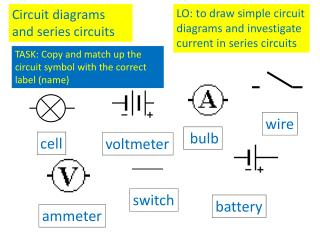DownloadDownload PresentationCircuit diagrams and series circuits

Circuit diagrams and series circuits

Télécharger la présentationCircuit diagrams and series circuits

- - - - - - - - - - - - - - - - - - - - - - - - - - - E N D - - - - - - - - - - - - - - - - - - - - - - - - - - -
Presentation Transcript

1. Circuit diagrams and series circuits LO: to draw simple circuit diagrams and investigate current in series circuits TASK: Copy and match up the circuit symbol with the correct label (name) wire bulb cell voltmeter switch battery ammeter

2. Current has only one path to flow through

3. Will these circuits work? Then copy and complete For a current to flow, the circuit must be 1. 2.

4. Current Electricity is the flow of electric charge, currentis the flow of e_______. The bigger the current, the m___ electrons are flowing around the circuit. The w___ carry the electrons from one terminal around the circuit to the other t______. Current is measure with an a_________ lamps

5. Then, complete Q2 on pg 30 and Q5 on pg 32 Use lines to connect the components and act as wires

6. Circuit 1 Circuit 2 Circuit 3 Q.Whatdo you notice about the current in each circuit? Does this tell you whether current is used up as it flows around the circuit? Current is ____ used up in a series circuit, it is the ______ in all parts of the circuit Make the following circuits and measure the current with the ammeter. The only difference between the circuits is the position of the ammeter. Record your results in a suitable table.

7. 2A 2A 2A 2A 2A 2A A SIMPLE CIRCUIT

8. Write a paragraph to explain what you have found out – use the questions below to help you. • Can you see any pattern in your results? • What do you notice as you increase the number of bulbs? • What do you notice as you increase the number of cells? Build the circuits with the correct number of components as shown in the table below. Look at the brightness of the bulbs and measure the current. Record your results in the table (use the words bright, dim and normal to describe the brightness of the bulbs).

9. If you add more cells to a circuit the bulbs will get brighter/dimmer because more/lesscurrent is flowing. If you add more bulbs to a circuit the bulbs will become brighter/dimmer because more/lesscurrent is flowing.

10. 1A 1A 1A 1A 1A 1A 1A A Series Circuit What happens now?

11. Lesson summary, discuss with your partner • What flows around a circuit? • What do we need for this to flow? • What pushes this around the circuit?

12. Electricity model • What forms the circuit in this model? • What goes round the circuit? • What represents energy in the circuit? • Where does the current collect energy? • Where does it give up energy? • In what ways is this model similar to your own ideas about electricity? In what ways is it different?